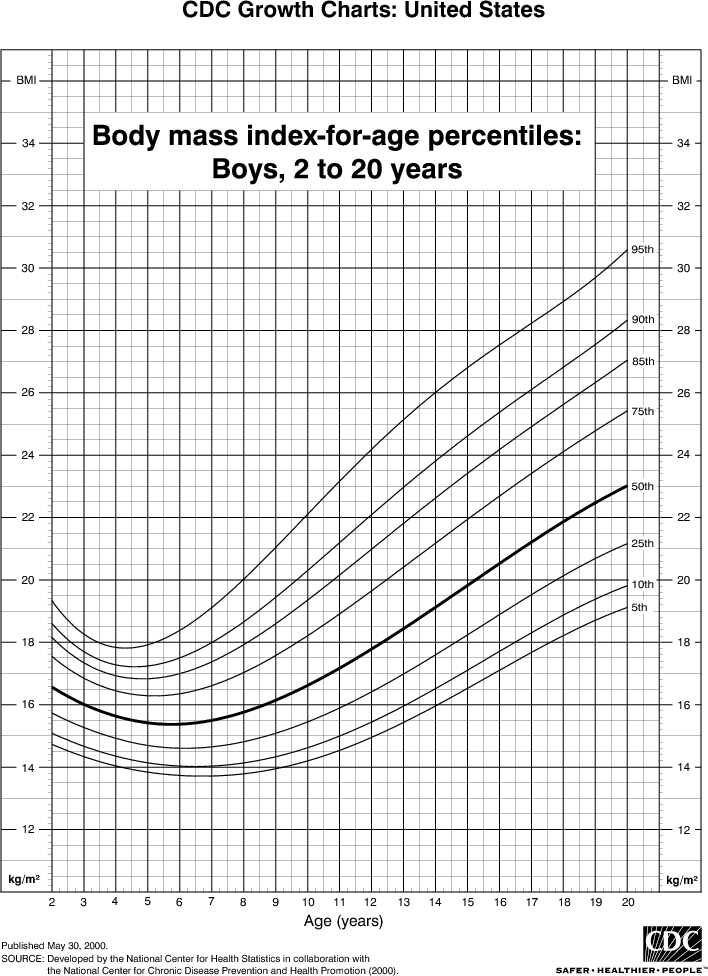Charts, Graphs, & Diagrams > Health Charts > Body Mass Index (BMI) Percentiles for Boys, 2 to 20 Years

## Body Mass Index (BMI) Percentiles for Boys, 2 to 20 Years

This is easy to use - no data input required. Find your child's BMI percentile in the chart based on height and weight.This chart shows the Body Mass Index (BMI) percentiles for boys from 2 to 20 years old.

#### How to read the percentile chart

The curved lines show BMI percentiles. For example, the top curved line shows the 95% percentile, which means that 95% of children are at or under that value.

The bottom of the chart shows ages, from 2 to 20 years. The left and right sides of the chart show BMI values.

The chart shows that at age 2 years 95% of boys have a BMI less than 19.4 and 5% have one less than 14.6. At 20 years 95% of boys have a BMI less than 30.6, and 5% have one less than 19.

#### How to find your child's BMI percentile

First, find your child's BMI in BMI for Children.

Then on this page, find your child's BMI on either side of the chart, and read horizontally across until you reach the vertical line for your child's age. Then see where that point is among the percentile lines.

For example, a boy with a BMI of 18 at age 12 is just above the 50% percentile.

#### Childrens weight status

The BMI for children is calculated the same way as for adults. However, it is interpreted differently. Here are the CDC weight status classifications for use with the above table:

PercentileWeight Status
95th-100thOverweight
85th-95thAt Risk of Overweight
5th-85thNormal
5th or lessUnderweight